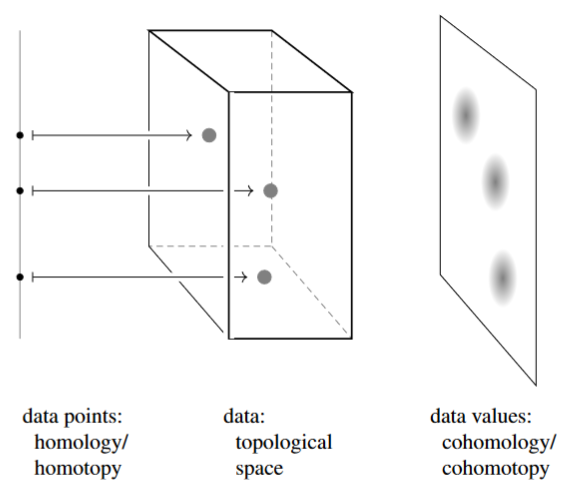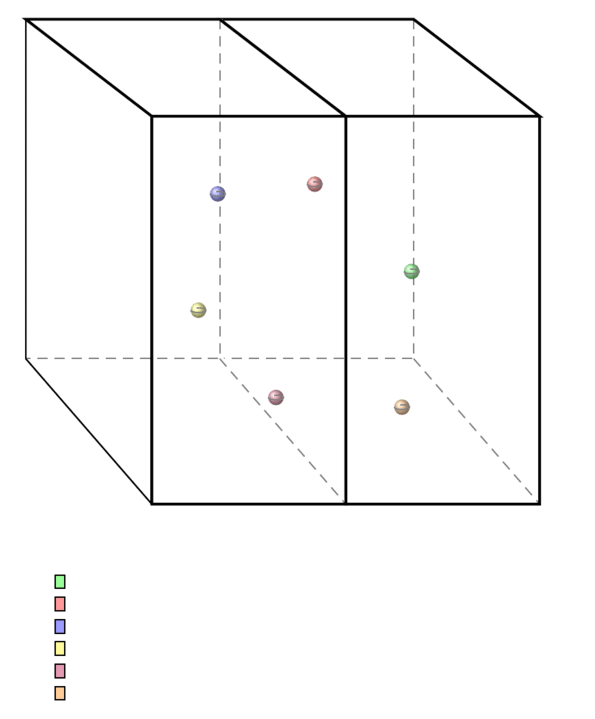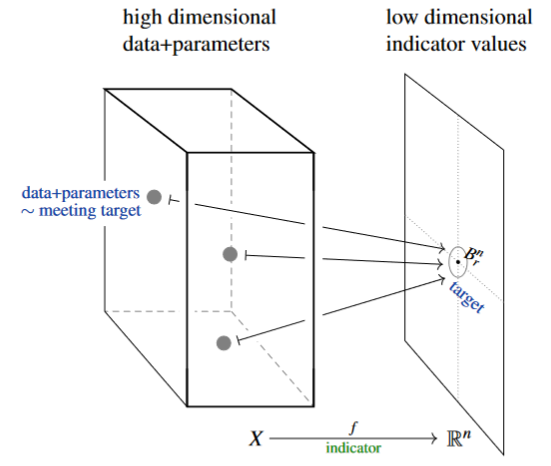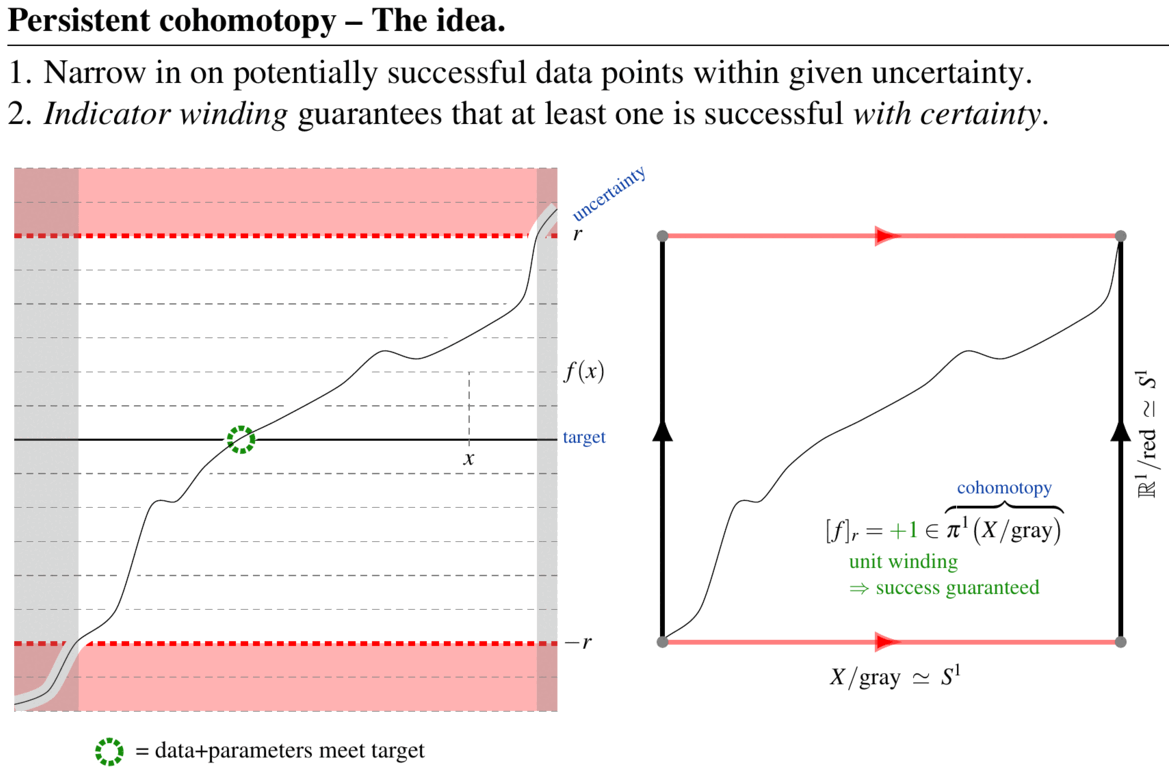# nLab topological data analysis

Topological data analysis

# Topological data analysis

## IdeaTopological data analysis (TDA) is qualitative data analysis with tools from topology, in particular with tools from algebraic topology, aiming to extract (hidden) structure in large datasets which is robust against uncertainties and noise.

This notably includes tools from ordinary (co-)homology-theory, which in the guise of persistent homology has become the signature method in TDA; but it also includes more general tools of homotopy theory and differential topology, which have more recently found their way into TDA in the guise of persistent homotopy theory and persistent cohomotopy theory. $\;\;\;\;\;\;\;$(graphics from SS22)

Typically, TDA deals with large data sets modeled as (subsets of) topological spaces. Collections of data points appear as cycles in the topological space of data, and values of data appear as cocycles.

### Strategy of persistent topology/homotopyThe strategy of persistent homology/homotopy in TDA is to see which chunks (subspaces) of the data appear to be (higher) connected when viewed at some resolution (technically: at some filter stage) and how much these apparent chunks persist as the resolution changes. This may be recorded in persistence diagrams also known as “barcodes”.

The fundamental theorem of the field – the stability theorem – says that persistent (co-)cycle-classes are indeed a good invariant of data, in that they remain stable under small perturbations of the initial data (e.g. under noise, uncertainty, measurement errors, etc.).

The idea then is that those (co-)cycles which persist for longer (appear as longer bars in the barcode) reflect relevant structure hidden in the (large) data set.

However, it is often unclear (and certainly not part of the mathematical theory) what significance or meaning any persistent cycle has for the practical problem of interpreting data. $\;\;\;\;\;\;$(graphics from SS22)

### Strategy of persistent cohomology/cohomotopyIn contrast, persistent cohomotopy in TDA is the effective answer to a concrete and common question in data analysis:

Given a large-dimensional space of data, and a small number $n$ of (real) indicator values assigned to each data point with given precision $1/r$, does any data meet a prescribed target indication precisely?

A fundamental theorem of persistent Cohomotopy (Franek, Krčál & Wagner 2018, Franek & Krčál 2017, p. 5, see here) shows that (1.) the answer to this question is detected by a certain Cohomotopy-class and (2.) in a fair range of dimensions, this Cohomotopy class is provably computable, hence the above question is effectively decidable. $\phantom{--}$ (graphics from SS22)(graphics from SS22)

(Alternatively, with tools from persistent homology theory an answer to this question is given by the method of well groups – but (1.) it is known that well groups are in general too coarse to provide a complete answer and (2.) despite effort it remains unknown if well groups are actually computable in relevant cases, see Franek & Krčál 2016.)

If the topological space $X$ of data may be assumed to be a smooth manifold (indeed, in typical examples $X$ is itself a large-dimensional Cartesian space) then persistent cohomotopy may be understood dually via Pontryagin's theorem as characterizing iso-hypersurfaces of data (close to a given target indicator) by framed cobordism theory (Franek & Krčál 2017, p. 8-9). The full implications of this relation for topological data analysis remain to be explored.

### General

General introduction and survey:

Flashy exposition for commercial use:

• Gunnar Carlsson, Professor Gunnar Carlsson Introduces Topological Data Analysis $[$video$]$

and see the references at

### Implementation

Implementation via concrete algorithms, etc.

Review:

• Stefan Huber, Persistent Homology in Data Science In: Data Science – Analytics and Applications Springer (2021) $[$doi:10.1007/978-3-658-32182-6_13, pdf$]$

### Applications

Application of topological data analysis (persistent homology) to

analysis of quasicrystals:

• Pavlo Solokha et al., New Quasicrystal Approximant in the Sc–Pd System: From Topological Data Mining to the Bench, Chem. Mater. 2020, 32, 3, 1064–1079 (doi:10.1021/acs.chemmater.9b03767)

analysis of cosmological structure formation:

analysis of phase transitions:

recognition of instantons and confinement in lattice gauge theory:

• Daniel Spitz, Julian M. Urban, Jan M. Pawlowski, Confinement in non-Abelian lattice gauge theory via persistent homology [arXiv:2208.03955]

analysis of the cosmic microwave background in search for hints of inhomogeneous cosmology:

Persistent homology has strong computational limits for large data sets and major problems with multifitrations. An alternative propoposal in TDA to persistent homology which is worse in 1 dimension but does not suffer above problems is

• Paweł Dłotko, Davide Gurnari, Euler Characteristic Curves and Profiles: a stable shape invariant for big data problems, arXiv:2212.01666